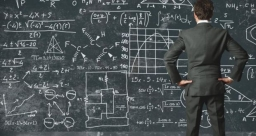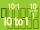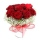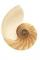# If-then equation

If 1/2 + 2/5s = s - 3/4

what is the value of s?

s =  2.0833

### Step-by-step explanation:Did you find an error or inaccuracy? Feel free to write us. Thank you!Tips to related online calculators
Need help to calculate sum, simplify or multiply fractions? Try our fraction calculator.
Do you have a linear equation or system of equations and looking for its solution? Or do you have a quadratic equation?

## Related math problems and questions:

• Equation with fractionsSolve an equation: 1- (2x-5)/(6) =3- (x)/(4) It is an equation with fractions.
• Two fraction equationFind d value of q in the equation 30/16=q/48.
• Equation with xSolve the following equation: 2x- (8x + 1) - (x + 2) / 5 = 9
• Two numbers 11The sum of two rational numbers is (-2). If one of them is 3/5, find the other.
• Equation with fractionsWhat is the simplified form of the equation fraction 4 over 5 n minus fraction 1 over 5 equals fraction 2 over 5 n? n = -2 n = 4 n = fraction 1 over 2
• Who IWho I am? My third is 2 less than my half.
• Two numbersThe sum of two numbers is 1. Identify this two numbers if you know that the half of first is equal to the third of second number.
• What is 9What is the value of x in the proportion 2 and one-fourth over x = 1 and one-half over 3 and three-fifths? 2 and two-fifths 5 and two-fifths 8 and 1 over 10 12 and 3 over 20
• The vaseThere were roses in the vase, half were red, a quarter was white and 12 were yellow. How many roses were in the vase?
• FractionsThree-quarters of an unknown number is 4/5. What is 5/6 of this unknown number?
• Over fractionSolve equation: p over 5 equals fraction numerator 7 p over denominator 6 end fraction plus 5
• Missing digitIf 4x/6x + 5/17 = 1, what digit does the x represents
• ExpressionIf it is true that (l + 15 w)/(w) = 6, then the value of the expression (13 l)/(12 w) is:
• Determine the numberDetermine the number x that ?.
• Equation with fractionsWhat is another way to write the equation 7/8 x + three-fourths = negative 6?
• Unknown numberIf I reduced the sum of the numbers 70 and the unknown number three times, I would get 100. what is the unknown number?
• Simple equation 324 = n • 27, solve for n